Theory and Modern Applications

# Boundary value problems for fractional differential equations with three-point fractional integral boundary conditions

## Abstract

This article studies a boundary value problem of nonlinear fractional differential equations with three-point fractional integral boundary conditions. Some new existence results are obtained by applying standard fixed point theorems. As an application, we give two examples that illustrate our results.

MSC:26A33, 34B15.

## 1 Introduction

Fractional differential equations have recently proved to be valuable tools in the modelling of many phenomena in various field of science and applications, such as physics, mechanics, chemistry, biology, economics, control theory, aerodynamics, engineering, etc. See . There has been a significant development in the theory of initial and boundary value problems for nonlinear fractional differential equations; see, for example, .

Ahmad and co-authors have studied the existence and uniqueness of solutions of nonlinear fractional differential and integro-differential equations for a variety of boundary conditions using standard fixed-point theorems and Leray-Schauder degree theory. Ahmad et al.  discusses the existence and uniqueness of solutions of fractional integro-differential equations for fractional nonlocal integral boundary conditions. Ahmad et al.  and references therein give details of recent work on the properties of solutions of sequential fractional differential equations. Ahmad et al.  considers solutions of fractional differential equations with non-separated type integral boundary conditions. In Ahmad et al. , the Krasnoselskii fixed point theorem and the contraction mapping principle are used to prove the existence of solutions of the nonlinear Langevin equation with two fractional orders for a number of different intervals. Ahmad et al.  discusses the existence and uniqueness of solutions of nonlinear fractional differential equations with three-point integral boundary conditions.

Cabada et al.  have also studied properties of solutions of nonlinear fractional differential equations. They used the properties of the associated Green’s function and the Guo-Krasnosellskii fixed-point theorem to investigate the existence of positive solutions of nonlinear fractional differential equations with integral boundary-value conditions.

Motivated by the papers  and , this article is concerned with the existence and uniqueness of solutions for a boundary value problem of nonlinear fractional differential equations with three-point fractional integral boundary conditions given by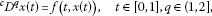(1.1)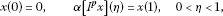(1.2)

where ${}^{c}D^{q}$ denotes the Caputo fractional derivative of order q, ${I}^{p}$ is the Riemann-Liouville fractional integral of order $p,f:\left[0,1\right]×\mathbb{R}\to \mathbb{R}$ is a continuous function and $\alpha \in \mathbb{R}$ is such that $\alpha \ne \mathrm{\Gamma }\left(p+2\right)/{\eta }^{p+1}$. By $C\left(\left[0,1\right],\mathbb{R}\right)$ we denote the Banach space of all continuous functions from $\left[0,1\right]$ into with the norm

$\parallel x\parallel =sup\left\{|x\left(t\right)|;t\in \left[0,1\right]\right\}.$

We note that if $p=1$, then condition (1.2) reduces to the usual three-point integral condition. In such a case, the boundary condition corresponds to the area under the curve of solutions $x\left(t\right)$ from $t=0$ to $t=\eta$.

## 2 Preliminaries

In this section, we introduce notations, definitions of fractional calculus and prove a lemma before stating our main results.

Definition 2.1 For a continuous function $f:\left[0,\mathrm{\infty }\right)\to \mathbb{R}$, the Caputo derivative of fractional order q is defined as

${}^{c}D^{q}f\left(t\right)=\frac{1}{\mathrm{\Gamma }\left(n-q\right)}{\int }_{0}^{t}{\left(t-s\right)}^{n-q-1}{f}^{\left(n\right)}\left(s\right)\phantom{\rule{0.2em}{0ex}}ds,\phantom{\rule{1em}{0ex}}n-1

provided that ${f}^{\left(n\right)}\left(t\right)$ exists, where $\left[q\right]$ denotes the integer part of the real number q.

Definition 2.2 The Riemann-Liouville fractional integral of order q for a continuous function $f\left(t\right)$ is defined as

${I}^{q}f\left(t\right)=\frac{1}{\mathrm{\Gamma }\left(q\right)}{\int }_{0}^{t}{\left(t-s\right)}^{q-1}f\left(s\right)\phantom{\rule{0.2em}{0ex}}ds,\phantom{\rule{1em}{0ex}}q>0,$

provided that such integral exists.

Definition 2.3 The Riemann-Liouville fractional derivative of order q for a continuous function $f\left(t\right)$ is defined by

${D}^{q}f\left(t\right)=\frac{1}{\mathrm{\Gamma }\left(n-q\right)}{\left(\frac{d}{dt}\right)}^{n}{\int }_{0}^{t}{\left(t-s\right)}^{n-q-1}f\left(s\right)\phantom{\rule{0.2em}{0ex}}ds,\phantom{\rule{1em}{0ex}}n=\left[q\right]+1,$

provided that the right-hand side is pointwise defined on $\left(0,\mathrm{\infty }\right)$.

Furthermore, we note that the Riemann-Liouville fractional derivative of a constant is usually nonzero which can cause serious problems in real would applications. Actually, the relationship between the two-types of fractional derivative is as follows

$\begin{array}{rcl}{}^{c}D^{q}f\left(t\right)& =& \frac{1}{\mathrm{\Gamma }\left(n-q\right)}{\int }_{0}^{t}\frac{{f}^{\left(n\right)}\left(s\right)}{{\left(t-s\right)}^{q+1-n}}\phantom{\rule{0.2em}{0ex}}ds\\ =& {D}^{q}f\left(t\right)-\sum _{k=0}^{n-1}\frac{{f}^{\left(k\right)}\left(0\right)}{\mathrm{\Gamma }\left(k-q+1\right)}{t}^{k-q}\\ =& {D}^{q}\left[f\left(t\right)-\sum _{k=0}^{n-1}\frac{{f}^{\left(k\right)}\left(0\right)}{k!}{t}^{k}\right],\phantom{\rule{1em}{0ex}}t>0,n-1

So, we prefer to use Caputo’s definition which gives better results than those of Riemann-Liouville.

Lemma 2.1 

Let $q>0$, then the fractional differential equation

${}^{c}D^{q}u\left(t\right)=0$

has solution

$u\left(t\right)={k}_{0}+{k}_{1}t+{k}_{2}{t}^{2}+\cdots +{k}_{n-1}{t}^{n-1},\phantom{\rule{1em}{0ex}}{k}_{i}\in \mathbb{R},i=0,1,2,\dots ,n-1,$

where n is the smallest integer greater than or equal to q.

Lemma 2.2 

Let $q>0$, then

${I}^{q}{\phantom{\rule{0.2em}{0ex}}}^{c}{D}^{q}u\left(t\right)=u\left(t\right)+{k}_{0}+{k}_{1}t+{k}_{2}{t}^{2}+\cdots +{k}_{n-1}{t}^{n-1},$

for some ${k}_{i}\in \mathbb{R}$, $i=0,1,2,\dots ,n-1$ where n is the smallest integer greater than or equal to q.

Lemma 2.3 Let $\alpha \ne \frac{\mathrm{\Gamma }\left(p+2\right)}{{\eta }^{p+1}}$, $1. Then for $h\in C\left(\left[0,1\right],\mathbb{R}\right)$, the problem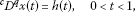(2.1)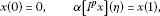(2.2)

has a unique solution

$\begin{array}{rcl}x\left(t\right)& =& \frac{1}{\mathrm{\Gamma }\left(q\right)}{\int }_{0}^{t}{\left(t-s\right)}^{q-1}h\left(s\right)\phantom{\rule{0.2em}{0ex}}ds-\frac{\mathrm{\Gamma }\left(p+2\right)t}{\mathrm{\Gamma }\left(q\right)\left(\mathrm{\Gamma }\left(p+2\right)-\alpha {\eta }^{p+1}\right)}{\int }_{0}^{1}{\left(1-s\right)}^{q-1}h\left(s\right)\phantom{\rule{0.2em}{0ex}}ds\\ +\frac{\alpha p\left(p+1\right)t}{\mathrm{\Gamma }\left(q\right)\left(\mathrm{\Gamma }\left(p+2\right)-\alpha {\eta }^{p+1}\right)}{\int }_{0}^{\eta }{\int }_{0}^{s}{\left(\eta -s\right)}^{p-1}{\left(s-r\right)}^{q-1}h\left(r\right)\phantom{\rule{0.2em}{0ex}}dr\phantom{\rule{0.2em}{0ex}}ds.\end{array}$
(2.3)

Proof By applying Lemma 2.2, we may reduce (2.1) to an equivalent integral equation

$x\left(t\right)=\frac{1}{\mathrm{\Gamma }\left(q\right)}{\int }_{0}^{t}{\left(t-s\right)}^{q-1}h\left(s\right)\phantom{\rule{0.2em}{0ex}}ds-{c}_{0}-{c}_{1}t,$
(2.4)

for some ${c}_{0},{c}_{1}\in \mathbb{R}$.

From $x\left(0\right)=0$, it follows ${c}_{0}=0$. Using the Riemann-Liouville integral of order p for (2.4), we have

$\begin{array}{rcl}{I}^{p}x\left(t\right)& =& \frac{1}{\mathrm{\Gamma }\left(p\right)}{\int }_{0}^{t}{\left(t-s\right)}^{p-1}\left[\frac{1}{\mathrm{\Gamma }\left(q\right)}{\int }_{0}^{s}{\left(s-r\right)}^{q-1}h\left(r\right)\phantom{\rule{0.2em}{0ex}}dr-{c}_{1}s\right]\phantom{\rule{0.2em}{0ex}}ds\\ =& \frac{1}{\mathrm{\Gamma }\left(p\right)\mathrm{\Gamma }\left(q\right)}{\int }_{0}^{t}{\int }_{0}^{s}{\left(t-s\right)}^{p-1}{\left(s-r\right)}^{q-1}h\left(r\right)\phantom{\rule{0.2em}{0ex}}dr\phantom{\rule{0.2em}{0ex}}ds-{c}_{1}\frac{{t}^{p+1}}{\mathrm{\Gamma }\left(p+2\right)}.\end{array}$

The second condition of (2.2) implies that

$\begin{array}{c}\frac{\alpha }{\mathrm{\Gamma }\left(p\right)\mathrm{\Gamma }\left(q\right)}{\int }_{0}^{\eta }{\int }_{0}^{s}{\left(\eta -s\right)}^{p-1}{\left(s-r\right)}^{q-1}h\left(r\right)\phantom{\rule{0.2em}{0ex}}dr\phantom{\rule{0.2em}{0ex}}ds-{c}_{1}\frac{\alpha {\eta }^{p+1}}{\mathrm{\Gamma }\left(p+2\right)}\hfill \\ \phantom{\rule{1em}{0ex}}=\frac{1}{\mathrm{\Gamma }\left(q\right)}{\int }_{0}^{1}{\left(1-s\right)}^{q-1}h\left(s\right)\phantom{\rule{0.2em}{0ex}}ds-{c}_{1}.\hfill \end{array}$

Thus,

$\begin{array}{rcl}{c}_{1}& =& \frac{\mathrm{\Gamma }\left(p+2\right)}{\mathrm{\Gamma }\left(q\right)\left(\mathrm{\Gamma }\left(p+2\right)-\alpha {\eta }^{p+1}\right)}{\int }_{0}^{1}{\left(1-s\right)}^{q-1}h\left(s\right)\phantom{\rule{0.2em}{0ex}}ds\\ -\frac{\alpha p\left(p+1\right)}{\mathrm{\Gamma }\left(q\right)\left(\mathrm{\Gamma }\left(p+2\right)-\alpha {\eta }^{p+1}\right)}{\int }_{0}^{\eta }{\int }_{0}^{s}{\left(\eta -s\right)}^{p-1}{\left(s-r\right)}^{q-1}h\left(r\right)\phantom{\rule{0.2em}{0ex}}dr\phantom{\rule{0.2em}{0ex}}ds.\end{array}$

Substituting the values of ${c}_{0}$ and ${c}_{1}$ in (2.4), we obtain the solution (2.3). □

In the following, for the sake of convenience, set

$\mathrm{\Lambda }=\frac{1}{\mathrm{\Gamma }\left(q+1\right)}+\frac{\mathrm{\Gamma }\left(p+2\right)}{\mathrm{\Gamma }\left(q+1\right)|\mathrm{\Gamma }\left(p+2\right)-\alpha {\eta }^{p+1}|}+\frac{\alpha {\eta }^{p+q}\mathrm{\Gamma }\left(p+2\right)}{\mathrm{\Gamma }\left(p+q+1\right)|\mathrm{\Gamma }\left(p+2\right)-\alpha {\eta }^{p+1}|}.$
(2.5)

## 3 Main results

Now we are in the position to establish the main results.

Theorem 3.1 Assume that there exists a constant $L>0$ such that

(${\mathrm{H}}_{1}$) $|f\left(t,x\right)-f\left(t,y\right)|\le L|x-y|$, for each $t\in \left[0,1\right]$, and all $x,y\in \mathbb{R}$.

If $L\mathrm{\Lambda }<1$, where Λ is defined by (2.5), then the BVP (1.1)-(1.2) has a unique solution on $\left[0,1\right]$.

Proof Transform the BVP (1.1)-(1.2) into a fixed point problem. In view of Lemma 2.3, we consider the operator $F:C\left(\left[0,1\right],\mathbb{R}\right)\to C\left(\left[0,1\right],\mathbb{R}\right)$ defined by

$\begin{array}{rcl}F\left(x\right)\left(t\right)& =& \frac{1}{\mathrm{\Gamma }\left(q\right)}{\int }_{0}^{t}{\left(t-s\right)}^{q-1}f\left(s,x\left(s\right)\right)\phantom{\rule{0.2em}{0ex}}ds\\ -\frac{\mathrm{\Gamma }\left(p+2\right)t}{\mathrm{\Gamma }\left(q\right)\left(\mathrm{\Gamma }\left(p+2\right)-\alpha {\eta }^{p+1}\right)}{\int }_{0}^{1}{\left(1-s\right)}^{q-1}f\left(s,x\left(s\right)\right)\phantom{\rule{0.2em}{0ex}}ds\\ +\frac{\alpha p\left(p+1\right)t}{\mathrm{\Gamma }\left(q\right)\left(\mathrm{\Gamma }\left(p+2\right)-\alpha {\eta }^{p+1}\right)}{\int }_{0}^{\eta }{\int }_{0}^{s}{\left(\eta -s\right)}^{p-1}{\left(s-r\right)}^{q-1}f\left(r,x\left(r\right)\right)\phantom{\rule{0.2em}{0ex}}dr\phantom{\rule{0.2em}{0ex}}ds.\end{array}$

Obviously, the fixed points of the operator F are solution of the problem (1.1)-(1.2). We shall use the Banach fixed point theorem to prove that F has a fixed point. We will show that F is a contraction.

Let $x,y\in C\left(\left[0,1\right],\mathbb{R}\right)$. Then, for each $t\in \left[0,1\right]$ we have

By using the property of beta function, $B\left(q+1,p\right)=\frac{\mathrm{\Gamma }\left(q+1\right)\mathrm{\Gamma }\left(p\right)}{\mathrm{\Gamma }\left(p+q+1\right)}$, we have

$\begin{array}{rcl}|F\left(x\right)\left(t\right)-F\left(y\right)\left(t\right)|& \le & \frac{L\parallel x-y\parallel }{\mathrm{\Gamma }\left(q+1\right)}+\frac{L\mathrm{\Gamma }\left(p+2\right)\parallel x-y\parallel }{\mathrm{\Gamma }\left(q+1\right)|\mathrm{\Gamma }\left(p+2\right)-\alpha {\eta }^{p+1}|}\\ +\frac{\alpha {\eta }^{p+q}\mathrm{\Gamma }\left(p+2\right)L\parallel x-y\parallel }{\mathrm{\Gamma }\left(p+q+1\right)|\mathrm{\Gamma }\left(p+2\right)-\alpha {\eta }^{p+1}|}.\end{array}$

Thus

$\parallel F\left(x\right)-F\left(y\right)\parallel \le L\mathrm{\Lambda }\parallel x-y\parallel .$

Therefore, F is a contraction. Hence, by Banach fixed point theorem, we get that F has a fixed point which is a solution of the problem (1.1)-(1.2). □

The following result is based on Schaefer’s fixed point theorem.

Theorem 3.2 Assume that:

(${\mathrm{H}}_{2}$) The function $f:\left[0,1\right]×\mathbb{R}\to \mathbb{R}$ is continuous.

(${\mathrm{H}}_{3}$) There exists a constant $M>0$ such that $|f\left(t,x\right)|\le M$ for each $t\in J$ and all $x\in \mathbb{R}$.

Then the BVP (1.1)-(1.2) has at least one solution on $\left[0,1\right]$.

Proof We shall use Schaefer’s fixed point theorem to prove that F has a fixed point. We divide the proof into four steps.

Step I. Continuity of F.

Let $\left\{{x}_{n}\right\}$ be a sequence such that ${x}_{n}\to x$ in $C\left(\left[0,1\right],\mathbb{R}\right)$. Then for each $t\in \left[0,1\right]$

$\begin{array}{c}|F\left({x}_{n}\right)\left(t\right)-F\left(x\right)\left(t\right)|\hfill \\ \phantom{\rule{1em}{0ex}}\le \frac{1}{\mathrm{\Gamma }\left(q\right)}{\int }_{0}^{t}{\left(t-s\right)}^{q-1}|f\left(s,{x}_{n}\left(s\right)\right)-f\left(s,x\left(s\right)\right)|\phantom{\rule{0.2em}{0ex}}ds\hfill \\ \phantom{\rule{2em}{0ex}}+\frac{\mathrm{\Gamma }\left(p+2\right)}{\mathrm{\Gamma }\left(q\right)|\mathrm{\Gamma }\left(p+2\right)-\alpha {\eta }^{p+1}|}{\int }_{0}^{1}{\left(1-s\right)}^{q-1}|f\left(s,{x}_{n}\left(s\right)\right)-f\left(s,x\left(s\right)\right)|\phantom{\rule{0.2em}{0ex}}ds\hfill \\ \phantom{\rule{2em}{0ex}}+\frac{\alpha p\left(p+1\right)}{\mathrm{\Gamma }\left(q\right)|\mathrm{\Gamma }\left(p+2\right)-\alpha {\eta }^{p+1}|}{\int }_{0}^{\eta }{\int }_{0}^{s}{\left(\eta -s\right)}^{p-1}{\left(s-r\right)}^{q-1}|f\left(r,{x}_{n}\left(r\right)\right)-f\left(r,x\left(r\right)\right)|\phantom{\rule{0.2em}{0ex}}dr\phantom{\rule{0.2em}{0ex}}ds\hfill \\ \phantom{\rule{1em}{0ex}}\le \frac{1}{\mathrm{\Gamma }\left(q\right)}{\int }_{0}^{t}{\left(t-s\right)}^{q-1}\underset{s\in \left[0,1\right]}{sup}|f\left(s,{x}_{n}\left(s\right)\right)-f\left(s,x\left(s\right)\right)|\phantom{\rule{0.2em}{0ex}}ds\hfill \\ \phantom{\rule{2em}{0ex}}+\frac{\mathrm{\Gamma }\left(p+2\right)}{\mathrm{\Gamma }\left(q\right)|\mathrm{\Gamma }\left(p+2\right)-\alpha {\eta }^{p+1}|}{\int }_{0}^{1}{\left(1-s\right)}^{q-1}\underset{s\in \left[0,1\right]}{sup}|f\left(s,{x}_{n}\left(s\right)\right)-f\left(s,x\left(s\right)\right)|\phantom{\rule{0.2em}{0ex}}ds\hfill \\ \phantom{\rule{2em}{0ex}}+\frac{\alpha p\left(p+1\right)}{\mathrm{\Gamma }\left(q\right)|\mathrm{\Gamma }\left(p+2\right)-\alpha {\eta }^{p+1}|}{\int }_{0}^{\eta }{\int }_{0}^{s}{\left(\eta -s\right)}^{p-1}{\left(s-r\right)}^{q-1}\hfill \\ \phantom{\rule{2em}{0ex}}×\underset{r\in \left[0,1\right]}{sup}|f\left(r,{x}_{n}\left(r\right)\right)-f\left(r,x\left(r\right)\right)|\phantom{\rule{0.2em}{0ex}}dr\phantom{\rule{0.2em}{0ex}}ds.\hfill \end{array}$

Since f is continuous function, then $\parallel F\left({x}_{n}\right)-F\left(x\right)\parallel \to 0$ as $n\to \mathrm{\infty }$. This means that F is continuous.

Step II. F maps bounded sets into bounded sets in $C\left(\left[0,1\right],\mathbb{R}\right)$.

So, let us prove that for any $r>0$, there exists a positive constant l such that for each $x\in {B}_{r}=\left\{x\in C\left(\left[0,1\right],\mathbb{R}\right):\parallel x\parallel \le r\right\}$, we have $\parallel F\left(x\right)\parallel \le l$. Indeed, we have for any $x\in {B}_{r}$

$\begin{array}{rcl}|F\left(x\right)\left(t\right)|& \le & \frac{1}{\mathrm{\Gamma }\left(q\right)}{\int }_{0}^{t}{\left(t-s\right)}^{q-1}|f\left(s,x\left(s\right)\right)|\phantom{\rule{0.2em}{0ex}}ds\\ +\frac{\mathrm{\Gamma }\left(p+2\right)}{\mathrm{\Gamma }\left(q\right)|\mathrm{\Gamma }\left(p+2\right)-\alpha {\eta }^{p+1}|}{\int }_{0}^{1}{\left(1-s\right)}^{q-1}|f\left(s,x\left(s\right)\right)|\phantom{\rule{0.2em}{0ex}}ds\\ +\frac{\alpha p\left(p+1\right)}{\mathrm{\Gamma }\left(q\right)|\mathrm{\Gamma }\left(p+2\right)-\alpha {\eta }^{p+1}|}{\int }_{0}^{\eta }{\int }_{0}^{s}{\left(\eta -s\right)}^{p-1}{\left(s-r\right)}^{q-1}|f\left(r,x\left(r\right)\right)|\phantom{\rule{0.2em}{0ex}}dr\phantom{\rule{0.2em}{0ex}}ds\end{array}$

which in view of (${\mathrm{H}}_{3}$) gives

$\begin{array}{rcl}|F\left(x\right)\left(t\right)|& \le & \frac{M}{\mathrm{\Gamma }\left(q\right)}{\int }_{0}^{t}{\left(t-s\right)}^{q-1}\phantom{\rule{0.2em}{0ex}}ds\\ +\frac{\mathrm{\Gamma }\left(p+2\right)M}{\mathrm{\Gamma }\left(q\right)|\mathrm{\Gamma }\left(p+2\right)-\alpha {\eta }^{p+1}|}{\int }_{0}^{1}{\left(1-s\right)}^{q-1}\phantom{\rule{0.2em}{0ex}}ds\\ +\frac{\alpha p\left(p+1\right)M}{\mathrm{\Gamma }\left(q\right)|\mathrm{\Gamma }\left(p+2\right)-\alpha {\eta }^{p+1}|}{\int }_{0}^{\eta }{\int }_{0}^{s}{\left(\eta -s\right)}^{p-1}{\left(s-r\right)}^{q-1}\phantom{\rule{0.2em}{0ex}}dr\phantom{\rule{0.2em}{0ex}}ds.\end{array}$

Hence, we deduce that

$\begin{array}{rcl}|F\left(x\right)\left(t\right)|& \le & \frac{M}{\mathrm{\Gamma }\left(q+1\right)}+\frac{\mathrm{\Gamma }\left(p+2\right)M}{\mathrm{\Gamma }\left(q+1\right)|\mathrm{\Gamma }\left(p+2\right)-\alpha {\eta }^{p+1}|}\\ +\frac{\alpha \mathrm{\Gamma }\left(p+2\right)M{\eta }^{p+q}}{\mathrm{\Gamma }\left(p+q+1\right)|\mathrm{\Gamma }\left(p+2\right)-\alpha {\eta }^{p+1}|}.\end{array}$

Thus,

$\parallel F\left(x\right)\parallel \le \mathrm{\Lambda }M:=l.$

Step III. We prove that $F\left({B}_{r}\right)$ is equicontinuous with ${B}_{r}$ defined as in Step II.

Let $0\le {t}_{1}<{t}_{2}\le 1$ and $x\in {B}_{r}$, then

$\begin{array}{c}|F\left(x\right)\left({t}_{2}\right)-F\left(x\right)\left({t}_{1}\right)|\hfill \\ \phantom{\rule{1em}{0ex}}=|\frac{1}{\mathrm{\Gamma }\left(q\right)}{\int }_{0}^{{t}_{1}}\left[{\left({t}_{2}-s\right)}^{q-1}-{\left({t}_{1}-s\right)}^{q-1}\right]f\left(s,x\left(s\right)\right)\phantom{\rule{0.2em}{0ex}}ds\hfill \\ \phantom{\rule{2em}{0ex}}+\frac{1}{\mathrm{\Gamma }\left(q\right)}{\int }_{{t}_{1}}^{{t}_{2}}{\left({t}_{2}-s\right)}^{q-1}f\left(s,x\left(s\right)\right)\phantom{\rule{0.2em}{0ex}}ds\hfill \\ \phantom{\rule{2em}{0ex}}-\frac{\left({t}_{2}-{t}_{1}\right)\mathrm{\Gamma }\left(p+2\right)}{\mathrm{\Gamma }\left(q\right)\left(\mathrm{\Gamma }\left(p+2\right)-\alpha {\eta }^{p+1}\right)}{\int }_{0}^{1}{\left(1-s\right)}^{q-1}f\left(s,x\left(s\right)\right)\phantom{\rule{0.2em}{0ex}}ds\hfill \\ \phantom{\rule{2em}{0ex}}+\frac{\alpha p\left(p+1\right)\left({t}_{2}-{t}_{1}\right)}{\mathrm{\Gamma }\left(q\right)\left(\mathrm{\Gamma }\left(p+2\right)-\alpha {\eta }^{p+1}\right)}{\int }_{0}^{\eta }{\int }_{0}^{s}{\left(\eta -s\right)}^{p-1}{\left(s-r\right)}^{q-1}f\left(r,x\left(r\right)\right)\phantom{\rule{0.2em}{0ex}}dr\phantom{\rule{0.2em}{0ex}}ds|\hfill \\ \phantom{\rule{1em}{0ex}}\le \frac{1}{\mathrm{\Gamma }\left(q\right)}{\int }_{0}^{{t}_{1}}\left[{\left({t}_{2}-s\right)}^{q-1}-{\left({t}_{1}-s\right)}^{q-1}\right]|f\left(s,x\left(s\right)\right)|\phantom{\rule{0.2em}{0ex}}ds\hfill \\ \phantom{\rule{2em}{0ex}}+\frac{1}{\mathrm{\Gamma }\left(q\right)}{\int }_{{t}_{1}}^{{t}_{2}}{\left({t}_{2}-s\right)}^{q-1}|f\left(s,x\left(s\right)\right)|\phantom{\rule{0.2em}{0ex}}ds\hfill \\ \phantom{\rule{2em}{0ex}}+\frac{\left({t}_{2}-{t}_{1}\right)\mathrm{\Gamma }\left(p+2\right)}{\mathrm{\Gamma }\left(q\right)|\mathrm{\Gamma }\left(p+2\right)-\alpha {\eta }^{p+1}|}{\int }_{0}^{1}{\left(1-s\right)}^{q-1}|f\left(s,x\left(s\right)\right)|\phantom{\rule{0.2em}{0ex}}ds\hfill \\ \phantom{\rule{2em}{0ex}}+\frac{\alpha p\left(p+1\right)\left({t}_{2}-{t}_{1}\right)}{\mathrm{\Gamma }\left(q\right)|\mathrm{\Gamma }\left(p+2\right)-\alpha {\eta }^{p+1}|}{\int }_{0}^{\eta }{\int }_{0}^{s}{\left(\eta -s\right)}^{p-1}{\left(s-r\right)}^{q-1}|f\left(r,x\left(r\right)\right)|\phantom{\rule{0.2em}{0ex}}dr\phantom{\rule{0.2em}{0ex}}ds\hfill \\ \phantom{\rule{1em}{0ex}}\le \frac{M}{\mathrm{\Gamma }\left(q\right)}{\int }_{0}^{{t}_{1}}\left[{\left({t}_{2}-s\right)}^{q-1}-{\left({t}_{1}-s\right)}^{q-1}\right]\phantom{\rule{0.2em}{0ex}}ds\hfill \\ \phantom{\rule{2em}{0ex}}+\frac{M}{\mathrm{\Gamma }\left(q\right)}{\int }_{{t}_{1}}^{{t}_{2}}{\left({t}_{2}-s\right)}^{q-1}\phantom{\rule{0.2em}{0ex}}ds\hfill \\ \phantom{\rule{2em}{0ex}}+\frac{\left({t}_{2}-{t}_{1}\right)\mathrm{\Gamma }\left(p+2\right)M}{\mathrm{\Gamma }\left(q\right)|\mathrm{\Gamma }\left(p+2\right)-\alpha {\eta }^{p+1}|}{\int }_{0}^{1}{\left(1-s\right)}^{q-1}\phantom{\rule{0.2em}{0ex}}ds\hfill \\ \phantom{\rule{2em}{0ex}}+\frac{\alpha p\left(p+1\right)\left({t}_{2}-{t}_{1}\right)M}{\mathrm{\Gamma }\left(q\right)|\mathrm{\Gamma }\left(p+2\right)-\alpha {\eta }^{p+1}|}{\int }_{0}^{\eta }{\int }_{0}^{s}{\left(\eta -s\right)}^{p-1}{\left(s-r\right)}^{q-1}\phantom{\rule{0.2em}{0ex}}dr\phantom{\rule{0.2em}{0ex}}ds\hfill \\ \phantom{\rule{1em}{0ex}}\le \frac{M}{\mathrm{\Gamma }\left(q+1\right)}\left[{\left({t}_{2}-{t}_{1}\right)}^{q}+\left({t}_{2}^{q}-{t}_{1}^{q}\right)\right]+\frac{M{\left({t}_{2}-{t}_{1}\right)}^{q}}{\mathrm{\Gamma }\left(q+1\right)}\hfill \\ \phantom{\rule{2em}{0ex}}+\frac{\left({t}_{2}-{t}_{1}\right)\mathrm{\Gamma }\left(p+2\right)M}{\mathrm{\Gamma }\left(q+1\right)|\mathrm{\Gamma }\left(p+2\right)-\alpha {\eta }^{p+1}|}\hfill \\ \phantom{\rule{2em}{0ex}}+\frac{\alpha {\eta }^{p+q}\left({t}_{2}-{t}_{1}\right)\mathrm{\Gamma }\left(p+2\right)M}{\mathrm{\Gamma }\left(p+q+1\right)|\mathrm{\Gamma }\left(p+2\right)-\alpha {\eta }^{p+1}|}.\hfill \end{array}$

Actually, as ${t}_{1}\to {t}_{2}$, the right-hand side of the above inequality tends to zero. As a consequence of Steps I to III together with the Arzela-Ascoli theorem, we get that $F:C\left(\left[0,1\right],\mathbb{R}\right)\to C\left(\left[0,1\right],\mathbb{R}\right)$ is completely continuous.

Step IV. A priori bounds.

We show that the set

is bounded.

Let $x\in E$. Then $x\left(t\right)=\lambda F\left(x\right)\left(t\right)$ for some $0<\lambda <1$. Thus, for each $t\in \left[0,1\right]$ we have

$\begin{array}{rcl}F\left(x\right)\left(t\right)& =& \frac{\lambda }{\mathrm{\Gamma }\left(q\right)}{\int }_{0}^{t}{\left(t-s\right)}^{q-1}f\left(s,x\left(s\right)\right)\phantom{\rule{0.2em}{0ex}}ds\\ -\frac{\lambda \mathrm{\Gamma }\left(p+2\right)t}{\mathrm{\Gamma }\left(q\right)\left(\mathrm{\Gamma }\left(p+2\right)-\alpha {\eta }^{p+1}\right)}{\int }_{0}^{1}{\left(1-s\right)}^{q-1}f\left(s,x\left(s\right)\right)\phantom{\rule{0.2em}{0ex}}ds\\ +\frac{\alpha \lambda p\left(p+1\right)t}{\mathrm{\Gamma }\left(q\right)\left(\mathrm{\Gamma }\left(p+2\right)-\alpha {\eta }^{p+1}\right)}{\int }_{0}^{\eta }{\int }_{0}^{s}{\left(\eta -s\right)}^{p-1}{\left(s-r\right)}^{q-1}f\left(r,x\left(r\right)\right)\phantom{\rule{0.2em}{0ex}}dr\phantom{\rule{0.2em}{0ex}}ds.\end{array}$

This implies by (${\mathrm{H}}_{3}$) that for all $t\in \left[0,1\right]$, we get

$\begin{array}{rcl}|F\left(x\right)\left(t\right)|& \le & \frac{\lambda M}{\mathrm{\Gamma }\left(q\right)}{\int }_{0}^{t}{\left(t-s\right)}^{q-1}\phantom{\rule{0.2em}{0ex}}ds\\ +\frac{\lambda \mathrm{\Gamma }\left(p+2\right)M}{\mathrm{\Gamma }\left(q\right)|\mathrm{\Gamma }\left(p+2\right)-\alpha {\eta }^{p+1}|}{\int }_{0}^{1}{\left(1-s\right)}^{q-1}\phantom{\rule{0.2em}{0ex}}ds\\ +\frac{\alpha \lambda p\left(p+1\right)M}{\mathrm{\Gamma }\left(q\right)|\mathrm{\Gamma }\left(p+2\right)-\alpha {\eta }^{p+1}|}{\int }_{0}^{\eta }{\int }_{0}^{s}{\left(\eta -s\right)}^{p-1}{\left(s-r\right)}^{q-1}\phantom{\rule{0.2em}{0ex}}dr\phantom{\rule{0.2em}{0ex}}ds\\ \le & \frac{M}{\mathrm{\Gamma }\left(q+1\right)}+\frac{\mathrm{\Gamma }\left(p+2\right)M}{\mathrm{\Gamma }\left(q+1\right)|\mathrm{\Gamma }\left(p+2\right)-\alpha {\eta }^{p+1}|}\\ +\frac{\alpha \mathrm{\Gamma }\left(p+2\right)M{\eta }^{p+q}}{\mathrm{\Gamma }\left(p+q+1\right)|\mathrm{\Gamma }\left(p+2\right)-\alpha {\eta }^{p+1}|}.\end{array}$

Hence, we deduce that

$\parallel F\left(x\right)\parallel \le \mathrm{\Lambda }M:=R.$

This shows that the set E is bounded. As a consequence of Schaefer’s fixed point theorem, we conclude that F has a fixed point which is a solution of the problem (1.1)-(1.2). □

## 4 Examples

In this section, in order to illustrate our results, we consider two examples.

Example 4.1 Consider the following three-point fractional integral boundary value problem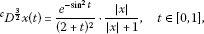(4.1)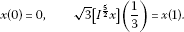(4.2)

Set $\eta =1/3$, $q=3/2$, $p=5/2$, $\alpha =\sqrt{3}\ne \mathrm{\Gamma }\left(p+2\right)/{\eta }^{p+1}=\mathrm{\Gamma }\left(9/2\right)/{\left(1/3\right)}^{\frac{7}{2}}$ and $f\left(t,x\right)=\left({e}^{-{sin}^{2}t}/{\left(2+t\right)}^{2}\right)\left(|x|/\left(1+|x|\right)\right)$. Since $\parallel f\left(t,x\right)-f\left(t,y\right)\parallel \le \left(1/4\right)\parallel x-y\parallel$, then, (${\mathrm{H}}_{1}$) is satisfied with $L=1/4$. We can show that

$\begin{array}{rcl}L\mathrm{\Lambda }& =& L\left[\frac{1}{\mathrm{\Gamma }\left(q+1\right)}+\frac{\mathrm{\Gamma }\left(p+2\right)}{\mathrm{\Gamma }\left(q+1\right)|\mathrm{\Gamma }\left(p+2\right)-\alpha {\eta }^{p+1}|}+\frac{\alpha {\eta }^{p+q}\mathrm{\Gamma }\left(p+2\right)}{\mathrm{\Gamma }\left(p+q+1\right)|\mathrm{\Gamma }\left(p+2\right)-\alpha {\eta }^{p+1}|}\right]\\ =& \frac{1}{4}\left[\frac{4}{3\sqrt{\pi }}+\frac{3\text{,}780}{2\text{,}835\sqrt{\pi }-16}+\frac{35\sqrt{3\pi }}{68\text{,}040\sqrt{\pi }-384}\right]\approx 0.376950576<1.\end{array}$

Hence, by Theorem 3.1, the boundary value problem (4.1)-(4.2) has a unique solution on $\left[0,1\right]$.

Example 4.2 Consider the following three-point fractional integral boundary value problem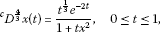(4.3)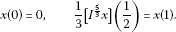(4.4)

Set $\eta =1/2$, $q=4/3$, $p=5/3$, $\alpha =1/3\ne \mathrm{\Gamma }\left(p+2\right)/{\eta }^{p+1}=\mathrm{\Gamma }\left(11/3\right)/{\left(1/2\right)}^{\frac{8}{3}}$ and $f\left(t,x\right)={t}^{\frac{1}{3}}{e}^{-2t}/\left(1+t{x}^{2}\right)$. Clearly $|f\left(t,x\right)|\le |{t}^{\frac{1}{3}}{e}^{-2t}/\left(1+t{x}^{2}\right)|\le 1=M$.

Hence, all the conditions of Theorem 3.2 are satisfied and consequently the problem (4.1)-(4.2) has at least one solution.

## References

1. Podlubny I: Fractional Differential Equations. Academic Press, San Diego; 1999.

2. Hilfer R: Fractional Calculus in Physics. World Scientific, Singapore; 2000.

3. Kilbas AA, Srivastava HM, Trujillo JJ: Theory and Applications of Fractional Differential Equations. Elsevier, Amsterdam; 2006.

4. Mainardi F: Fractional Calculus and Waves in Linear Viscoelasticity: An Introduction to Mathematical Models. Imperial College Press, Singapore; 2010.

5. Ortigueira MD: Fractional Calculus for Scientists and Engineers. Heidelberg, Springer; 2011.

6. Petras I: Fractional-Order Nonlinear Systems: Modeling, Analysis and Simulation. Springer, Berlin; 2011.

7. Ahmad B: Existence of solutions for irregular boundary value problems of nonlinear fractional differential equations. Appl. Math. Lett. 2010, 23: 390–394. 10.1016/j.aml.2009.11.004

8. Ahmad B, Sivasundaram S: On four-point nonlocal boundary value problems of nonlinear integro-differential equations of fractional order. Appl. Math. Comput. 2010, 217: 480–487. 10.1016/j.amc.2010.05.080

9. Bai Z, Qiu T: Existence of positive solution for singular fractional differential equation. Appl. Math. Comput. 2009, 215: 2761–2767. 10.1016/j.amc.2009.09.017

10. Delbosco D, Rodino L: Existence and uniqueness for a nonlinear fractional differential equation. J. Math. Anal. Appl. 1996, 204: 609–625. 10.1006/jmaa.1996.0456

11. Deng J, Ma L: Existence and uniqueness of solutions of initial value problems for nonlinear fractional differential equations. Appl. Math. Lett. 2010, 23: 676–680. 10.1016/j.aml.2010.02.007

12. Han X, Wang T: The existence of solutions for a nonlinear fractional multi-point boundary value problem at resonance. Int. J. Differ. Equ. 2011., 2011: Article ID 401803

13. Jiang W: The existence of solutions to boundary value problems of fractional differential equations at resonance. Nonlinear Anal. 2011, 74: 1987–1994. 10.1016/j.na.2010.11.005

14. Rehman MU, Khan RA: Existence and uniqueness of solutions for multi-point boundary value problems for fractional differential equations. Appl. Math. Lett. 2010, 23: 1038–1044. 10.1016/j.aml.2010.04.033

15. Wang G, Liu W, Yang J, Zhu S, Zheng T: The existence of solutions for a fractional 2 m -point boundary value problems. J. Appl. Math. 2012., 2012: Article ID 841349

16. Ahmad B, Nieto JJ: Riemann-Liouville fractional integro-differential equations with fractional nonlocal integral conditions. Bound. Value Probl. 2011., 2011: Article ID 36

17. Ahmad, B, Nieto, JJ: Sequential fractional differential equations with three-point boundary conditions. Comput. Math. Appl. (in press). http://dx.doi.org/10.1016/j.camwa.2012.02.036

18. Ahmad B, Nieto JJ, Alsaedi A: Existence and uniqueness of solutions for nonlinear fractional differential equations with non-separated type integral boundary conditions. Acta Math. Sci., Ser. B 2011, 31(6):2122–2130. 10.1016/S0252-9602(11)60388-3

19. Ahmad B, Nieto JJ, Alsaedi A, El-Shahed M: A study of nonlinear Langevin equation involving two fractional orders in different intervals. Nonlinear Anal., Real World Appl. 2012, 13: 599–606. 10.1016/j.nonrwa.2011.07.052

20. Ahmad B, Ntouyas SK, Alsaedi A: New existence results for nonlinear fractional differential equations with three-point integral boundary conditions. Adv. Differ. Equ. 2011., 2011: Article ID 107384

21. Cabada A, Wang G: Positive solutions of nonlinear fractional differential equations with integral boundary value conditions. J. Math. Anal. Appl. 2012, 389: 403–411. 10.1016/j.jmaa.2011.11.065

## Acknowledgements

The authors thank the referees for several useful remarks and interesting comments. This research is supported by the Centre of Excellence in Mathematics, the Commission on Higher Education, Thailand.

## Author information

Authors

### Competing interests

The authors declare that they have no competing interests.

### Authors’ contributions

All authors contributed equally and significantly in writing this article. All authors read and approved the final manuscript.

## Rights and permissions

Reprints and Permissions

Sudsutad, W., Tariboon, J. Boundary value problems for fractional differential equations with three-point fractional integral boundary conditions. Adv Differ Equ 2012, 93 (2012). https://doi.org/10.1186/1687-1847-2012-93

• Accepted:

• Published:

• DOI: https://doi.org/10.1186/1687-1847-2012-93

### Keywords

• existence
• Caputo fractional derivative
• Riemann-Liouville fractional integral
• boundary value problem